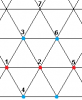BrainDen.com - Brain Teasers
• 0

# Monochromatic equilateral triangle on a 2-colored plane

## Question

I have found recent puzzles here to be as delightfully counter-intuitive as others that deal with probability and infinity. They are the puzzles where each point on a line, a figure, and here the entire plane, receives a specific color. The notion of an isolated point (described by a pair of real numbers) is beyond common experience and sometimes requires proficiency in real number theory to work with. We may wonder what an isolated point actually is, and whether it has "adjacent" points. Nevertheless intuition can sometimes lead us to say, without rigor, that something must be or can't be true.

Example

For every 2-coloring of the plane (arbitrarily assign to every point (x, y) a red or blue color,) will there always be a unit equilateral triangle whose vertices are monochromatic?

## Recommended Posts

• 0

No. Color the plane so that (x,y) is red if floor(2y/sqrt3) is even, and blue if it is odd. In other words, the plane is colored in horizontal stripes, each stripe just short of being able to fit a unit equilateral triangle.

Consider the y-distance between two vertices of the triangle. If it is less than sqrt(3)/2, the two vertices can be of the same color only if they are in the same stripe. If it is equal to sqrt(3)/2, they can never be of the same color. If it is greater than sqrt(3)/2, they can be the same color only if they are in different stripes. Each triangle's vertices yield three pairs of points. If one pair of vertices in a unit equilateral triangle (say AB) has a y-distance greater than sqrt(3)/2, then the other two pairs (AC and BC) have y-distance less than sqrt(3)/2. So A must be in the same stripe as C, which must be in the same stripe as B, but A must be in a different stripe from B. This is a contradiction. Conversely, if two pairs of vertices have y-distance less than sqrt(3)/2, then the third pair must have y-distance greater than sqrt(3)/2, which leads us to the same contradiction. So no unit equilateral triangle can have monochromatic vertices for this coloring.

##### Share on other sites

• 0

Yes. Here is why...

Choose an arbitrary point, an arbitrary distance d, and an arbitrary direction. This establishes a triangular grid of points equidistant from their "neighbors". Let's attempt to paint the vertices of this grid with 2 colors in a way that there are no monochromatic equilateral triangles.Take the first 3 points that are neighbors. Two of them will have to be of the same color. WLOG, say points #1 and #2 are red and #3 is blue. Then we must paint point #4 blue to avoid a monochromatic triangle (1,2,4). Since points 3, 4 and 5 create an equilateral triangle, point 5 must be red. So, then point 6 must be blue. Now, look at point #7. If it's red it will for a red triangle (1,5,7). If it's blue, then triangle (3,6,7) is blue.

##### Share on other sites

• 0

Can you ensure, for every coloring, that a monochromatic triangle with sides of unit length can be found?

Note that a simple scaling changes the coloring.

## Join the conversation

You can post now and register later. If you have an account, sign in now to post with your account.×   Pasted as rich text.   Paste as plain text instead

Only 75 emoji are allowed.

×   Your previous content has been restored.   Clear editor

×   You cannot paste images directly. Upload or insert images from URL.